+91-8872006993, +91-8872006994, +91-8872006995
Maths Lab

 Maths LabMath Lab at Green Land School provides unique opportunity to students to understand, internalize discover & verify the basic mathematical & geometrical concepts .Math Lab is helpful in building interest & confidence in students for learning the mathematical concepts in easiest way.

Advantages of Math Lab to Students:
1. The Math Lab helps the students to verify or discover several geometrical properties & facts.
2. Using tools like ‘The geometers sketchpad’ the students will be able to create an object & explore its mathematical properties through and exiting visual process, a process that would help in building a strong foundation in Euclidean & non – Euclidean geometry.
3. Math lab provides greater scope for individual participation in the process of learning.
4. Math Lab helps in developing the habit of verifications in the students. They accept mathematical truths only after testing their validity practically.

Close

SST Lab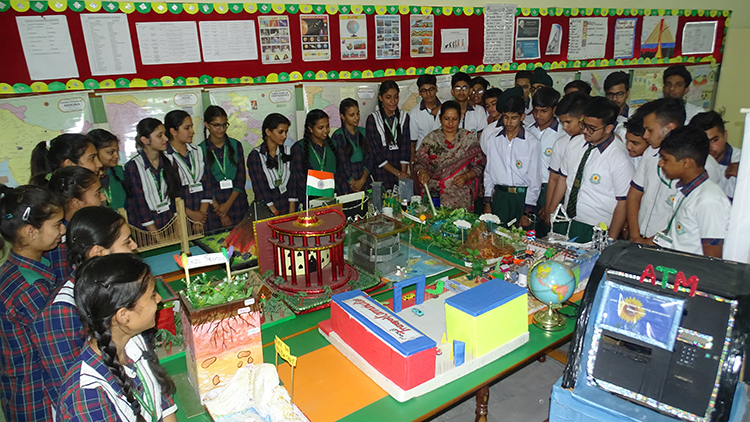Close

Dance Room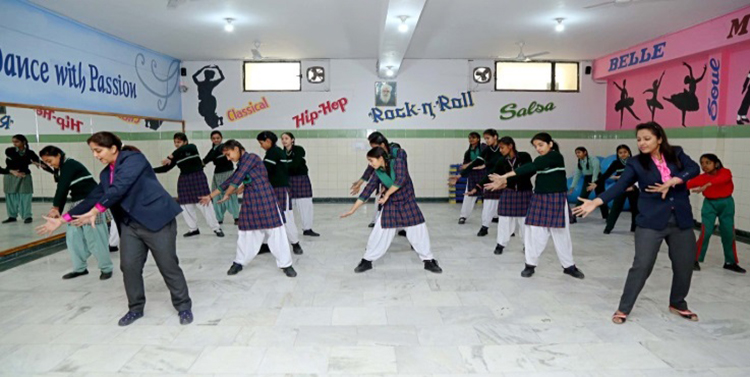Close

Computer Lab

 Computer LabIn Green Land School there is facility of computer lab to all its students. Computer Lab is beneficial to students in terms of learning the basic concepts of computer’s and their operation. The students can implement the theoretical concepts being taught in class in to practical.

Close

Science Labs

 Chemistry Lab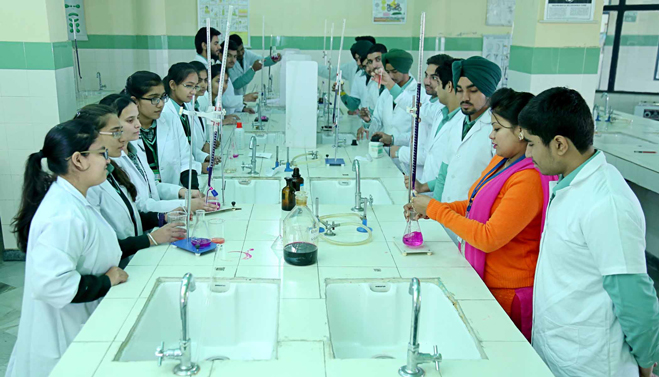Green Land School provides separate Physics, Chemistry and Biology labs to students. All the labs are well equipped with the instruments where the students can perform the experiments. All the labs are spacious and well ventilated. Labs are equipped with first-aid to handle any accidental situation.

 Biology LabClose

Toy Room

 Toy Room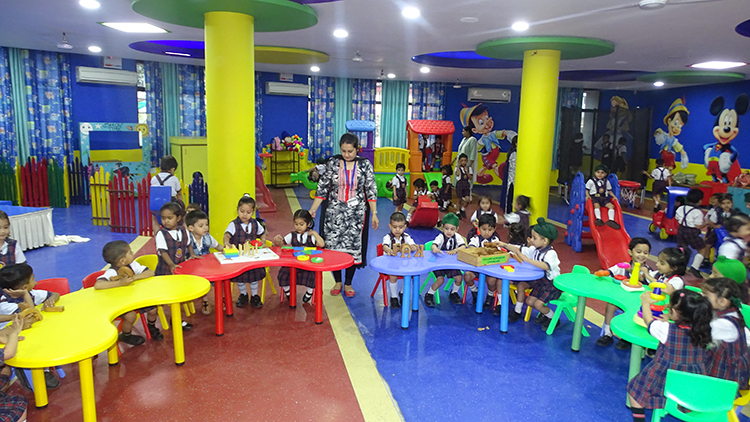Green Land School Dugri provides the facility of Toy Room to its students.

Close

Library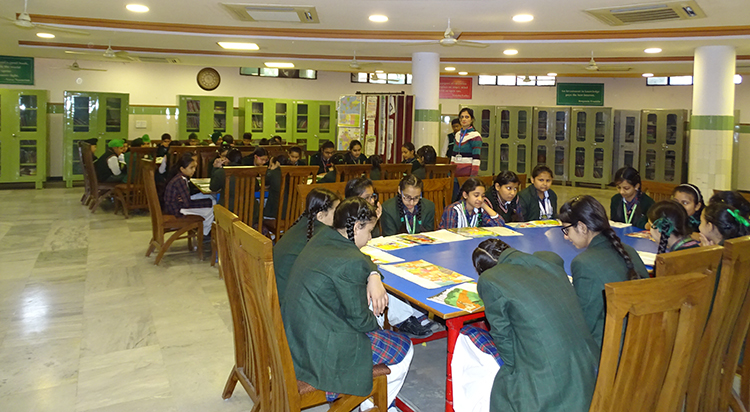Close

Smart ClassClose

Music Room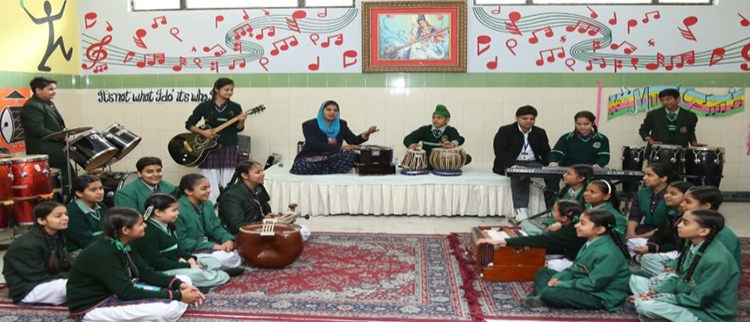Close

English Language LabClose